The VARMAX Procedure

Tentative Order Selection

Subsections:

Sample Cross-Covariance and Cross-Correlation Matrices

Given a stationary multivariate time series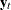, cross-covariance matrices are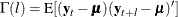where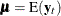, and cross-correlation matrices are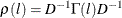where D is a diagonal matrix with the standard deviations of the components ofon the diagonal.

The sample cross-covariance matrix at lag l, denoted as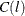, is computed as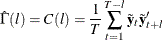where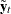is the centered data and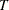is the number of nonmissing observations. Thus,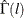has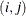th element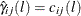. The sample cross-correlation matrix at lag l is computed as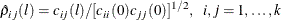The following statements use the CORRY option to compute the sample cross-correlation matrices and their summary indicator plots in terms of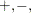and, where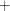indicates significant positive cross-correlations,indicates significant negative cross-correlations, andindicates insignificant cross-correlations.

proc varmax data=simul1;
model y1 y2 / p=1 noint lagmax=3 print=(corry)
printform=univariate;
run;


Figure 35.39 shows the sample cross-correlation matrices of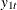and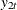. As shown, the sample autocorrelation functions for each variable decay quickly, but are significant with respect to two standard errors.

Figure 35.39: Cross-Correlations (CORRY Option)

The VARMAX Procedure

Cross Correlations of Dependent Series
by Variable
Variable Lag y1 y2
y1 0 1.00000 0.67041
1 0.83143 0.84330
2 0.56094 0.81972
3 0.26629 0.66154
y2 0 0.67041 1.00000
1 0.29707 0.77132
2 -0.00936 0.48658
3 -0.22058 0.22014

Schematic Representation
of Cross Correlations
Variable/Lag 0 1 2 3
y1 ++ ++ ++ ++
y2 ++ ++ .+ -+

Partial Autoregressive Matrices

For each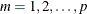you can define a sequence of matrices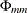, which is called the partial autoregression matrices of lag m, as the solution forto the Yule-Walker equations of order m,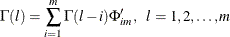The sequence of the partial autoregression matricesof order m has the characteristic property that if the process follows the AR(p), then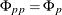and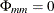for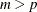. Hence, the matriceshave the cutoff property for a VAR(p) model, and so they can be useful in the identification of the order of a pure VAR model.

The following statements use the PARCOEF option to compute the partial autoregression matrices:

proc varmax data=simul1;
model y1 y2 / p=1 noint lagmax=3
printform=univariate
print=(corry parcoef pcorr
pcancorr roots);
run;


Figure 35.40 shows that the model can be obtained by an AR order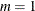since partial autoregression matrices are insignificant after lag 1 with respect to two standard errors. The matrix for lag 1 is the same as the Yule-Walker autoregressive matrix.

Figure 35.40: Partial Autoregression Matrices (PARCOEF Option)

The VARMAX Procedure

Partial Autoregression
Lag Variable y1 y2
1 y1 1.14844 -0.50954
y2 0.54985 0.37409
2 y1 -0.00724 0.05138
y2 0.02409 0.05909
3 y1 -0.02578 0.03885
y2 -0.03720 0.10149

Schematic Representation
of Partial Autoregression
Variable/Lag 1 2 3
y1 +- .. ..
y2 ++ .. ..

Partial Correlation Matrices

Define the forward autoregression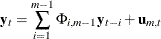and the backward autoregression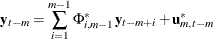The matricesdefined by Ansley and Newbold (1979) are given by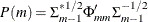where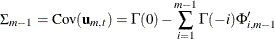and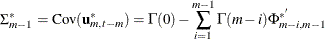are the partial cross-correlation matrices at lag m between the elements of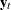and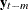, given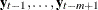. The matriceshave the cutoff property for a VAR(p) model, and so they can be useful in the identification of the order of a pure VAR structure.

The following statements use the PCORR option to compute the partial cross-correlation matrices:

proc varmax data=simul1;
model y1 y2 / p=1 noint lagmax=3
print=(pcorr)
printform=univariate;
run;


The partial cross-correlation matrices in Figure 35.41 are insignificant after lag 1 with respect to two standard errors. This indicates that an AR order ofcan be an appropriate choice.

Figure 35.41: Partial Correlations (PCORR Option)

The VARMAX Procedure

Partial Cross Correlations by Variable
Variable Lag y1 y2
y1 1 0.80348 0.42672
2 0.00276 0.03978
3 -0.01091 0.00032
y2 1 -0.30946 0.71906
2 0.04676 0.07045
3 0.01993 0.10676

Schematic Representation
of Partial Cross
Correlations
Variable/Lag 1 2 3
y1 ++ .. ..
y2 -+ .. ..

Partial Canonical Correlation Matrices

The partial canonical correlations at lag m between the vectorsand, given, are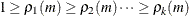. The partial canonical correlations are the canonical correlations between the residual series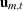and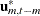, whereandare defined in the previous section. Thus, the squared partial canonical correlations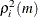are the eigenvalues of the matrix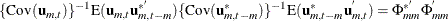It follows that the test statistic to test for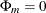in the VAR model of orderis approximately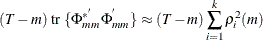and has an asymptotic chi-square distribution with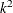degrees of freedom for.

The following statements use the PCANCORR option to compute the partial canonical correlations:

proc varmax data=simul1;
model y1 y2 / p=1 noint lagmax=3 print=(pcancorr);
run;


Figure 35.42 shows that the partial canonical correlations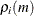betweenandare {0.918, 0.773}, {0.092, 0.018}, and {0.109, 0.011} for lags1 to 3. After lag1, the partial canonical correlations are insignificant with respect to the 0.05 significance level, indicating that an AR order ofcan be an appropriate choice.

Figure 35.42: Partial Canonical Correlations (PCANCORR Option)

The VARMAX Procedure

Partial Canonical Correlations
Lag Correlation1 Correlation2 DF Chi-Square Pr > ChiSq
1 0.91783 0.77335 4 142.61 <.0001
2 0.09171 0.01816 4 0.86 0.9307
3 0.10861 0.01078 4 1.16 0.8854

The Minimum Information Criterion (MINIC) Method

The minimum information criterion (MINIC) method can tentatively identify the orders of a VARMA(p,q) process (Spliid, 1983; Koreisha and Pukkila, 1989; Quinn, 1980). The first step of this method is to obtain estimates of the innovations series,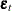, from the VAR(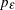), whereis chosen sufficiently large. The choice of the autoregressive order,, is determined by use of a selection criterion. From the selected VAR() model, you obtain estimates of residual series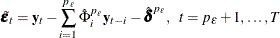In the second step, you select the order (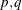) of the VARMA model for p in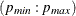and q in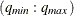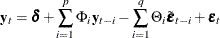which minimizes a selection criterion like SBC or HQ.

The following statements use the MINIC= option to compute a table that contains the information criterion associated with various AR and MA orders:

proc varmax data=simul1;
model y1 y2 / p=1 noint minic=(p=3 q=3);
run;


Figure 35.43 shows the output associated with the MINIC= option. The criterion takes the smallest value at AR order 1.

Figure 35.43: MINIC= Option

The VARMAX Procedure

Minimum Information Criterion Based on AICC
Lag MA 0 MA 1 MA 2 MA 3
AR 0 3.3574947 3.0331352 2.7080996 2.3049869
AR 1 0.5544431 0.6146887 0.6771732 0.7517968
AR 2 0.6369334 0.6729736 0.7610413 0.8481559
AR 3 0.7235629 0.7551756 0.8053765 0.8654079